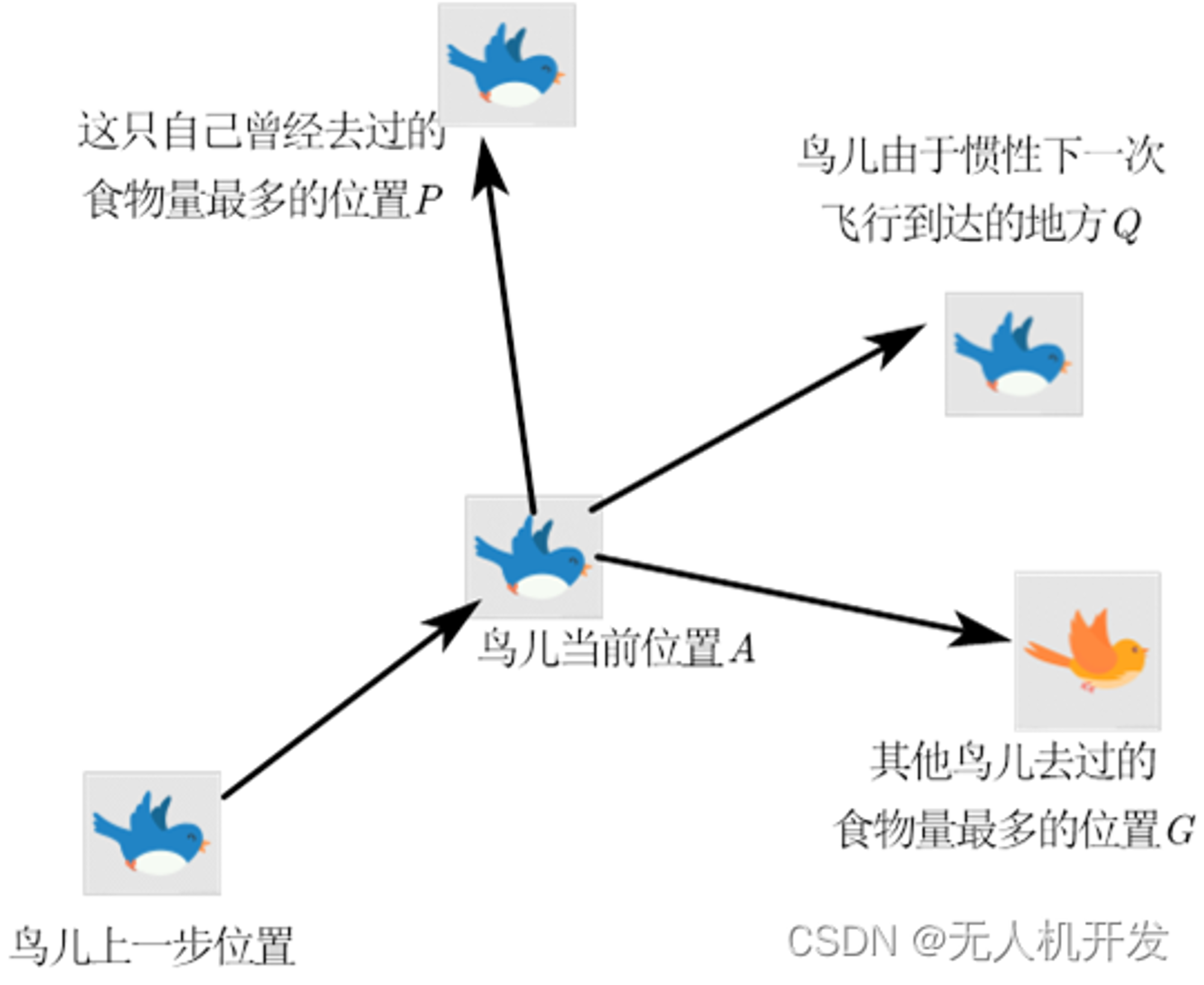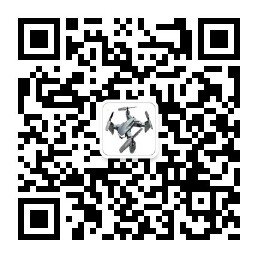V1

2022/11/19阅读：42主题：默认主题

# 粒子群算法及其改进

## 2、粒子群算法介绍在粒子群优化算法中，每个解可用一只鸟（粒子）表示，目标函数就是鸟群所需要寻找的食物源。寻找最优解的过程中，粒子包含两种行为：个体行为和群体行为。

## 3、各种粒子群算法改进

1、将学习因子自适应话； 2、引入遗传算法特性，与之进行结合； 3、结合混沌特性，使其不易陷入局部最优； 4、二阶粒子群算法 5、与模拟退火算法结合； 6、二阶振荡粒子群算法

## 4、部分代码及仿真结果

for t=1:M    for i=1:N        w = wmax - (t-1)*(wmax-wmin)/(M-1);        v(i,:)=w*v(i,:)+c1*rand*(y(i,:)-x(i,:))+c2*rand*(pg-x(i,:));        x(i,:)=x(i,:)+v(i,:);        if fitness(x(i,:))<p(i)            p(i)=fitness(x(i,:));            y(i,:)=x(i,:);        end        if p(i)<fitness(pg)            pg=y(i,:);        end    end    Pbest(t)=fitness(pg);end

## 关注公众号，获取全部改进代码

❤️ 关注我领取海量matlab电子书和相关资料

❤️ 扫一扫，添加我的公众号或者搜索【无人机开发】V1